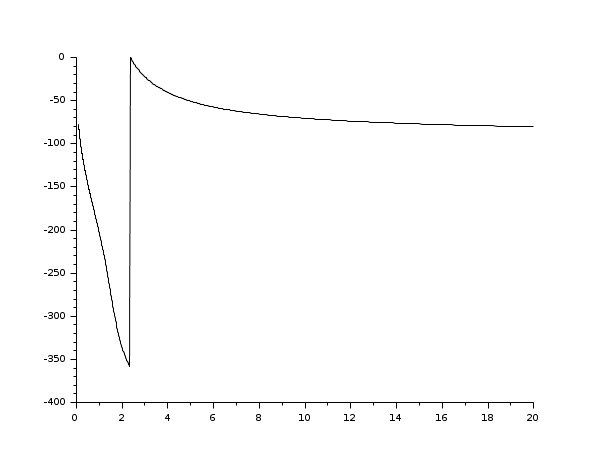Scilab Home page | Wiki | Bug tracker | Forge | Mailing list archives | ATOMS | File exchange
Change language to: Français - Português - 日本語 - Русский

See the recommended documentation of this function

# phasemag

phase and magnitude computation

### Calling Sequence

`[phi,db]=phasemag(z [,mod])`

### Arguments

z

matrix or row vector of complex numbers.

mod

character string

mod='c'

"continuous" representation between -infinity and +360 degrees (default)

mod='m'

representation between -360 and 0 degrees

phi

phases (in degree) of `z`.

db

magnitude (in Db)

### Description

`phasemag` computes the phases and magnitudes of the entries of a complex matrix. For `mod='c'``phasemag` computes `phi(:,i+1)` to minimize the distance with `phi(:,i)`, i.e. it tries to obtain a "continuous representation" of the phase.

To obtain the phase between -%pi and %pi use `phi=atan(imag(z),real(z))`

### Examples

```s=poly(0,'s');
h=syslin('c',1/((s+5)*(s+10)*(100+6*s+s*s)*(s+.3)));
[frq,rf]=repfreq(h,0.1,20,0.005);
scf();
plot2d(frq',phasemag(rf,'c')');
scf();
plot2d(frq',phasemag(rf,'m')');```• repfreq — frequency response
• gainplot — magnitude plot